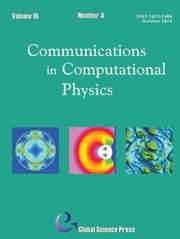Home
Hostname: page-component-5c569c448b-nqqt6 Total loading time: 0.272 Render date: 2022-07-04T08:56:39.350Z Has data issue: true Feature Flags: { "shouldUseShareProductTool": true, "shouldUseHypothesis": true, "isUnsiloEnabled": true, "useRatesEcommerce": false, "useNewApi": true } hasContentIssue trueCommunications in Computational Physics

# Stochastic Multi-Symplectic Integrator for Stochastic Nonlinear Schrödinger Equation

Published online by Cambridge University Press:  03 June 2015

*

## Abstract

In this paper we propose stochastic multi-symplectic conservation law for stochastic Hamiltonian partial differential equations, and develop a stochastic multi-symplectic method for numerically solving a kind of stochastic nonlinear Schrödinger equations. It is shown that the stochastic multi-symplectic method preserves the multi-symplectic structure, the discrete charge conservation law, and deduces the recurrence relation of the discrete energy. Numerical experiments are performed to verify the good behaviors of the stochastic multi-symplectic method in cases of both solitary wave and collision.

Type
Research Article
Information
Communications in Computational Physics , August 2013 , pp. 393 - 411

## Access options

Get access to the full version of this content by using one of the access options below. (Log in options will check for institutional or personal access. Content may require purchase if you do not have access.)

## References

Bang, O., Christiansen, P.L., ø, K.Rasmussen, White noise in the two-dimensional nonlinear Schrödinger equation, Appl. Math., 57 (1995), 315.Google Scholar
Bouard, A.De, Debussche, A., Di Menza, L., Theoretical and numerical aspects of stochastic nonlinear Schrödinger equations, Monte-Carlo Meth. Appl., 7(1-2)(2001), 5563.CrossRefGoogle Scholar
Debussche, A., Di Menza, L., Numerical simulation of focusing stochastic nonlinear Schrödinger equations, Phys. D,162(2002), 131154.CrossRefGoogle Scholar
De Bouard, A., Debussche, A., Weak and strong order of convergence of a semi discrete scheme for the stochastic Nonlinear Schrodinger equation, Appl. Mathe. Optim., 54 (2006), 369399.CrossRefGoogle Scholar
Abdullaev, F.Kh., Garuier, J., Soliton in media with random dispersive perturbations, Physica. D, 134 (1999), 303315.CrossRefGoogle Scholar
Bridges, T., Reich, S., Multi-symplectic integrators: numerical schemes for Hamiltonian PDEs that conserve symplecticity, Phys. Lett. A, 284 (2001), 184193.CrossRefGoogle Scholar
Hairer, E., Lubich, C., Wanner, G., Geometric Numerical Integration, Structure-Preserving Algorithms for Ordinary Differential Equations, Springer-Verlag, 2002.Google Scholar
Hong, J., Liu, Y., H Munthe-Kaas and A Zanna, Globally conservative properties and error estimation of a multi-symplectic scheme for Schrödinger equations with variable coefficients, Appl. Numer. Math., 56 (2006), 814843.CrossRefGoogle Scholar
Hong, J., Liu, X., Li, C., Multi-symplectic Runge-Kutta methods for nonlinear Schrödinger equations with variable coefficients, J. Comput. Phys., 226 (2007), 19681984.CrossRefGoogle Scholar
Hong, J., Scherer, R., Wang, L., Midpoint Rule for a Linear Stochastic Oscillator with Additive Noise, Neural Parallel and Scientific Computing, 14 (2006), 112.Google Scholar
Iserles, A., A First Course in the Numerical Analysis of Differential Equations, Cambridge University Press, Cambridge, 1996.Google Scholar
Islas, A., Karpeev, D., Schober, C., Geometric integrators for the nonlinear Schrödinger equation, J. Comput. Phys., 173 (2001), 116148.CrossRefGoogle Scholar
Konotop, V., Vazquez, L., Nonlinear Random Waves, World Scientific, River Edge. NJ, 1994.CrossRefGoogle Scholar
Marsden, J., Patrick, G., Shkoller, S., Multi-symplectic geometry, variational integrators, and nonlinear PDEs, Comm. Math. Phys. 199(1998), 351395.CrossRefGoogle Scholar
Milstein, G., Tretyakov, M., Stochastic Numerics for Mathematical Physics, Kluwer Academic Publishers, 1995.Google Scholar
Rasmussen, k. ø., Gaididei, Y.B., Bang, O., Christiansen, P.L., The influence of noise on critical collapse in the nonlinear Schrödinger equation, Phys. Rev. A, 204 (1995), 121127.Google Scholar
Schober, C., Symplectic integrators for the Ablowitz-Ladik discrete nonlinear Schrödinger equation, Phys. Lett. A, 259 (1999), 140151.CrossRefGoogle Scholar
Shardlow, T., Weak convergence of a numerical method for a stochastic heat equation, BIT, 43 (2003), 179193.CrossRefGoogle Scholar
19
Cited by

# Save article to Kindle

Note you can select to save to either the @free.kindle.com or @kindle.com variations. ‘@free.kindle.com’ emails are free but can only be saved to your device when it is connected to wi-fi. ‘@kindle.com’ emails can be delivered even when you are not connected to wi-fi, but note that service fees apply.

Find out more about the Kindle Personal Document Service.

Stochastic Multi-Symplectic Integrator for Stochastic Nonlinear Schrödinger Equation
Available formats
×

# Save article to Dropbox

To save this article to your Dropbox account, please select one or more formats and confirm that you agree to abide by our usage policies. If this is the first time you used this feature, you will be asked to authorise Cambridge Core to connect with your Dropbox account. Find out more about saving content to Dropbox.

Stochastic Multi-Symplectic Integrator for Stochastic Nonlinear Schrödinger Equation
Available formats
×

# Save article to Google Drive

To save this article to your Google Drive account, please select one or more formats and confirm that you agree to abide by our usage policies. If this is the first time you used this feature, you will be asked to authorise Cambridge Core to connect with your Google Drive account. Find out more about saving content to Google Drive.

Stochastic Multi-Symplectic Integrator for Stochastic Nonlinear Schrödinger Equation
Available formats
×
×